##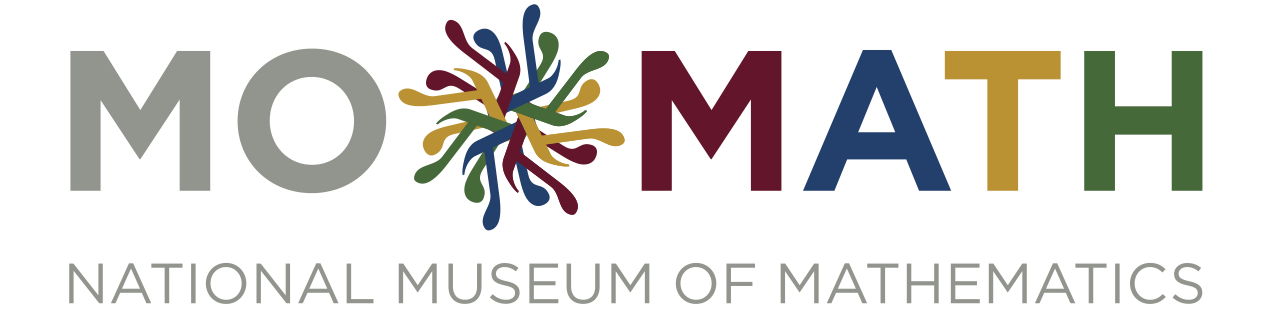With the year drawing to a close, time seems to be on everyone’s mind, and that’s reflected in today’s lineup.
[asciimathsf]

### Champion Countdown

To close out the year, Skyler is planning to count down from 2015 to 0, ending by finishing saying “Zero!” at precisely midnight on the evening of 2015 Dec 31. This will of course take careful planning, so Skyler has done some timings. The four-digit numbers take four seconds to say, the three-digit numbers three seconds to say, the two digit numbers two seconds, and the one-digit numbers one second. Also, as a result of careful breathing exercises, Skyler only needs to pause after the first 100 numbers he says, and after each subsequent 100 numbers. Each pause lasts exactly five seconds, and otherwise Skyler says all of the numbers without any interruption or time between the numbers.

Three questions for this puzzle: (A) Precisely what time/date should Skyler start saying “2015”? (B) 2015 seconds after Skyler starts, what number is recited? (C) 2015 seconds before midnight on December 31, what number is recited? If in either (B) or (C) Skyler is precisely between numbers at the instant mentioned, your answer should be the next number Skyler recites; otherwise it should be the number Skyler is in the midst of saying.

### Crooked Clock

The clock in the Varsity Math training room is an ordinary analog 12-hour clock with three hands. Each of the hour, minute and second hands sweeps around the clock continuously, making one revolution per twelve hours, one hour and one minute, respectively. And as usual, they are all aligned pointing perfectly straight up at noon and midnight each day.

How many times per day are there simultaneously two pairs of hands that make a perfect right angle with each other?

## Solutions to Week 13

Tee Party. First, choose the five fonts for the first occurrence of each letter. This can be done in 7×6×5×4×3 ways. Now, how many ways are there to choose the fonts for the three repeated letters? They could all three be repeated fonts (1 option), or you could choose one not to repeat and choose a new font for it (3 letters × 2 fonts = 6 options) or you could choose one to repeat and then assign the two remaining fonts to the two other letters (3 letters × 2 assignments = 6 options). Since you can’t choose new fonts for all three repeated letters (there aren’t enough fonts), that’s all the options for the repeated letters. Hence, there are a total of 13×7×6×5×4×3 = 32760 font designs for the team t-shirts.

Slingshot Grid. From the first three digits of the previous answer, we know there are 327 points. The only non-trivial rectangle that has 327 dots is 3×109, since 3 and 109 are prime. Thus, the dots range from (0,0) to (108,2). Next, we determine that it is impossible to slingshot beyond x = 324. Let the three added points be P, Q and R, in that order. If Q is produced using P as one of the slingshot points, then R cannot be added using the line through P and Q (since all of the slingshot lines must be different), so therefore the slingshot line for R must include two points from the original rectangle, and therefore its interval cannot be longer than 108 units in the x direction, and so R could not possibly have x-coordinate greater than three times 108, or 324. On the other hand, if P and Q were each produced from the original rectangle of dots, then their x-coordinates would have to be between -54 (the farthest-left initial slingshot, using points with x-coordinates 108, 54 and 0) and 162 (the farthest-right initial slingshot, using points with x-coordinates 0, 54, and 108). So in this case, the farthest right R could be is 162 + (162 + 54)/2 = 270.

Finally, we just need to see that we can achieve an x-coordinate of 324: Slingshot (0,2), (48,2) and (96,2) to produce (144,2). Then slingshot (0,0), (72,1) and (144,2) to produce (216,3). Finally, slingshot (0,1), (108,2), and (216,3) to produce (324,4), as desired. Note that all three lines are different because they all have different y-intercepts—we used all three points with x = 0, once each.

Try Angles. The first diagram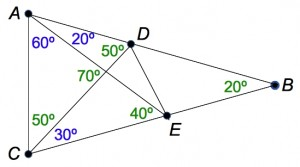shows all of the angles filled in from the answers to the prior problems (in blue) and a number of other angles (in green) easily determined from them using the fact that triangle △ABC is isosceles and that the angle sum of a triangle is 180°. Unfortunately, none of these is the desired angle ∠DEA.

To determine that angle, let P be the point on segment AE such that distance AP = AC. Then because ∠PAC = 60°, △PAC is equilateral and PC = AC.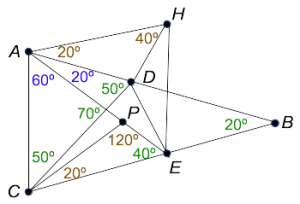The angles of △PCE can be easily determined, as shown in brown. Since △CAD is isosceles, AD = AC = PC, so we can erect a copy △ADH of △PCE on segment AD. Since ∠HAC = 100° (the supplement of ∠ACE) and AH = CE by construction, quadrilateral ACEH is a parallelogram. This fact tells us that ∠AHE = 80° and ∠HEC = 100°, so ∠AEH = 60°. Now evidently AD bisects ∠EAH and HD bisects ∠AHE. But the point of intersection of two angle bisectors of a triangle (here △AHE, and see the Coach’s notes) bisects the third angle. Hence DE bisects ∠AEH and DEA = 30°.

Coach Newton’s notes —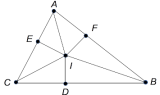There are many beautiful and surprising facts about even such a simple-seeming mathematical structure as a triangle. For this problem, we used the principle that the angle bisectors of any triangle are concurrent, which just means that all three of them intersect in a single point. To see why that’s true, imagine any triangle △ABC and let I be the intersection of two of the angle bisectors. Drop perpendiculars from I to all three sides. If I bisects ∠BAC, say, then △AFI is congruent to △AEI so FI = EI. Because of the other angle bisection, another pair of the perpendiculars are analogously equal. In other words, DI = EI = FI, and all three angles are bisected.

Great Lengths. Let `JG=x` and `FG=y`, so that we are looking for a triangle with side lengths `x-y`, `x`, and `x+y` which has one angle equal to twice another. Note as stipulated that `x` and `y` must be integers; and we are looking for the shortest side `x-y` to be of minimum length.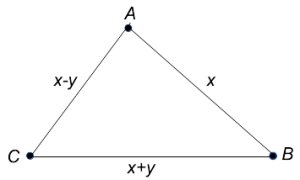It’s reasonable to guess that the angle at point `A` (as in the diagram) opposite the longest side `x+y` will be twice the angle at `B` opposite the shortest side `x-y`. Applying the law of cosines to each angle, we have `(x+y)^2 = (x-y)^2 + x^2 − 2(x-y)(x)cos A` and `(x-y)^2 = (x+y)^2 + x^2 − 2(x+y)(x)cos B`. The double angle formula tells us that `cos A = 2cos^2 B – 1`, so we solve the second equation for `cos B = (x + 4y)/(2(x+y))` and substitute into the first to get `2(x-y)(x)(2((x + 4y)/(2(x+y)))^2 – 1) = x^2 – 4xy`. We can now cancel a factor of `x` and multiply both sides by `2(x+y)^2` to get `2(x-y)((x + 4y)^2 – 2(x+y)^2) = 2(x-4y)(x+y)^2`. Multiplying out and collecting terms all on one side, we have `4x^3-14x^2y=34xy^2+20y^3=0`. Dividing by `2y^3`, this is `2(x/y)^3 – 7(x/y)^2-17(x/y)+10=0`, which factors as `(x/y – 5)(2(x/y)^2+3(x/y)-2)=0`. So one possibility is that `x/y=5`, and since `x` and `y` must be whole numbers, the minimum possibility is `x=5` and `y=1`. Hence, `x-y=4` and we know that a 4,5,6 triangle has one angle twice another.

All that remains is to check whether any smaller value of `x-y` will work. Since `y>=1`, `x-y` cannot be 1, because the three sides will not form a triangle (the triangle inequality will not be satisfied). If `x-y=2`, the only possibility is a 2,3,4 triangle, and if `x-y=3`, the only possibilities are a 3,4,5 triangle and a 3,5,7 triangle. It is easy to check that none of these three triangles has one angle twice another, for example by using the law of cosines again. Therefore, the smallest side length possible is 4.

## Recent Weeks

Links to all of the puzzles and solutions are on the Complete Varsity Math page.

Come back next week for answers and more puzzles.